Life

Calculate how many years has man lived for 12248 days?

Result

n =  33

Solution:Leave us a comment of this math problem and its solution (i.e. if it is still somewhat unclear...):Be the first to comment!Next similar math problems:

1. Book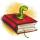Louise read from their favorite book for three days 18 pages. Every day read the same number of pages. How many pages she read every day?
2. Workshop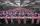In the workshop have to be produced for 5 days 2000 components. How many components must be produced in the workshop every day and how much per hour, if shift is 8 hours.
3. Bakers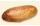Baker Martin baked 5 times more cakes than Dennis. How many cakes baked Dennis if Martin bake 25 cakes?
4. Multiples of int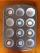How many multiples of 3 is between the numbers to 20? (zero not count)
5. Crates 2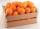One crate will hold 50 oranges. If Bob needs to ship 932 oranges, how many crates will he need?
6. Cars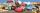Johnny has 370 cars. Peter twice less. How many cars have Peter?
7. Pimps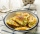There were 24 pimps in the plate. Maros ate 12, his little sister four times less. How many pimps remained in the plate?
8. Like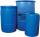When is in 14 barrels 140 liters of water, how many liters barrels of 8 liters I need to get all the water from the larger barrels?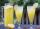How many 1/4 cup servings are in 2 and 1/3 cups of lemonade?
10. A koala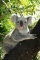A koala lives to be 14 years old in the wild. How much longer will a 2 year old koala probably live?
11. Salary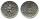Mr. Vesely got for work 874 CZK (Czech Republic Koruna). Mr. Jaros got twice less than Mr. Vesely. How much CZK got Mr. Jaros?
12. Dividing by five and tenNumber 5040 divide by the number 5 and by number 10: a = 5040: 5 b = 5040: 10
13. Flour2 kg of flour costs 100 CZK. How much does it cost half a kilogram?
14. BreederSeven hens breeder has supplied 350 eggs. How long can deliver, if each of its chickens can withstand at least 5 eggs for week?
15. Pitcher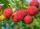Matthew picked up half-pitcher of raspberry in 45 minutes. Find how long three children filled the pitcher if each of them working at the same pace as Matthew.
16. Three numbers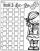How much we increases the sum of three numbers when the first enlarge by 14, second by 15 and third by 16? Choose any three two-digit numbers and prove results.
17. Unknown number 22I am a two-digit number. My tens digits is 4. My ones digit is 3 more than my tens. Who am I?